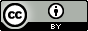Notes on variables

Contents

things to talk about eventually:

• $$p \leftrightarrow q$$ vs $$p \equiv q$$.
• Propositional functions: view them as $$f: \{\mathrm{T},\mathrm{F}\}^n\to \{\mathrm{T},\mathrm{F}\}$$? The tautologies are all $$f$$ such that for any $$x\in \{\mathrm{T},\mathrm{F}\}^n$$, we have $$f(x) = \mathrm{T}$$?

Now, for some $$x\in \{\mathrm{T},\mathrm{F}\}^n$$, what do we make of the statement $$f(x)$$? Is it true or false?

• bound variables in expressions like $$\int_a^b f(x)\, dx$$ and $$\sum_{i=0}^n f(i)$$ vs in ones like $$\exists x P(x)$$

• why different results for partial and total derivative? cf. Tao’s analysis book

Tao’s example: take $$f$$ defined by $$f(x,y) = (x^2, y^2)$$. Then $$\displaystyle \frac{\partial f}{\partial x} = (2x,0)$$, whereas $$\displaystyle \frac{d}{dx}f(x,x) = (2x,2x)$$.

• transfer pdf notes

• what do we make of statements like “when $$x=3$$…”?

• How do we make sense of things like the partial derivative, where one variable is “moving” while the others stay “constant”?

• “as $$x$$ gets larger…”

• $$a_n \to \infty$$ as $$n\to 5$$

• $$ax + b = 0$$

Concepts to discuss:

• parameter, arbitrary fixed constant, , flowing letters, meta-variables
• distinction between bound universals and free arbitrary variables
• undefined/unspecified/unknown/undetermined
• known constants vs unknown constants
• undefined constants vs variables
• declaration of variable type
• variable assignment
• here is an example of when variables can lead to confusion.
• temporary (new) constants

Mathematicians and computer scientists are usually not careful with a function versus the output of a function. So for instance when using the big-Oh notation, people will write $$\mathcal{O}(n)$$ (which is imprecise, because it doesn’t specify what the input variable is; is $$n$$ the parameter or a constant?) instead of “$$\mathcal{O}(f)$$, where $$f(n) = n$$” or “$$\mathcal{O}(\lambda n.n)$$”.

Similarly, when dealing with Laplace transforms, it seems common to write both $$\mathcal{L}\{f(t)\} = F(s)$$ and $$\mathcal{L}\{f\} = F(s)$$; but $$s$$ is not present on the left hand side of either denotation! To be pedantic, we would need to write $$\mathcal{L}\{f\} = F$$ or $$\mathcal{L}\{f\}(s) = F(s)$$ or $$\mathcal{L}\{\lambda t.f(t)\}(s) = F(s)$$.

In differential equations, it also seems common to write something like $y'' + p(t)y' + q(t)y = f(t)$ but $$y$$ is a function depending on $$t$$, so shouldn’t it instead be the following? $y''(t) + p(t)y'(t) + q(t)y(t) = f(t)$ Or more simply $y'' + py' + qy = f$

Questions

• What is the difference between a parameter and a variable?
• What is the difference between a variable and a meta-variable?
• In expressions like $$ax + b$$, is there an essential difference between $$x$$ and the other letters, even when we say that $$x$$ is a variable while $$a$$ and $$b$$ are constants?
• What does it mean to define some variable $$y$$ as a function of another variable $$x$$?
• e.g. what does it mean to say something like “as $$y$$ gets larger, $$x$$ also gets larger”?
• Two possible interpretations: (1) Treat $$y = f(x)$$ as a condition, and look at different possibilities like “if $$x = 1$$, then $$y = f(1)$$”, and so on. (2) treat $$y$$ as a machine that outputs different things for different inputs. So as $$x$$ is “adjusted”, $$y$$ is affected too.